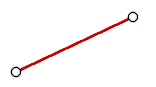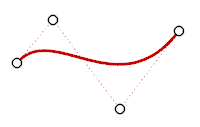### Contents

1. Getting Started with Modeling
1. Curve Degree
2.  Commands
1. 2D Modeling
2. 3D Modeling
3. Set Operations
4. Translation and Measurement

### Getting Started with Modeling

#### Curve Degree

The degree is a index to evaluate curvature. The higher the degree of the curve is, the smoother the curvature of the curve is. The degree is mathematic term and basically indicates the order of the polynomial.

That is to say, “degree 1 continuity” means that the curve is continuous in zeroth-order differentiation (= continuous in position), “degree 2 continuity” means that the curve is continuous in first-order differentiation (=continuous in tangent), and “degree 3 continuity” means that the curve is continuous in second-order differentiation (=continuous in curvature).degree 1 : line, polylinedegree 2 : parabola, arc, circledegree 3 : curve